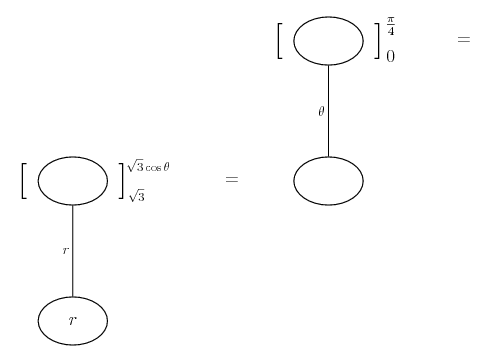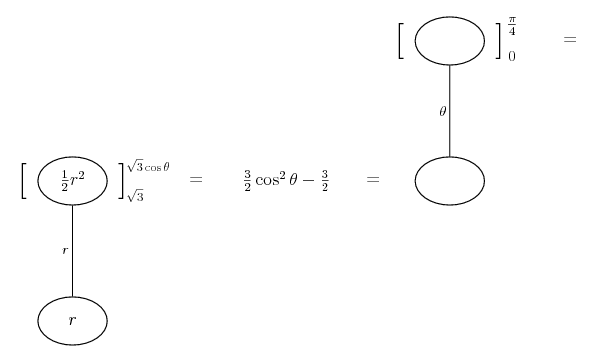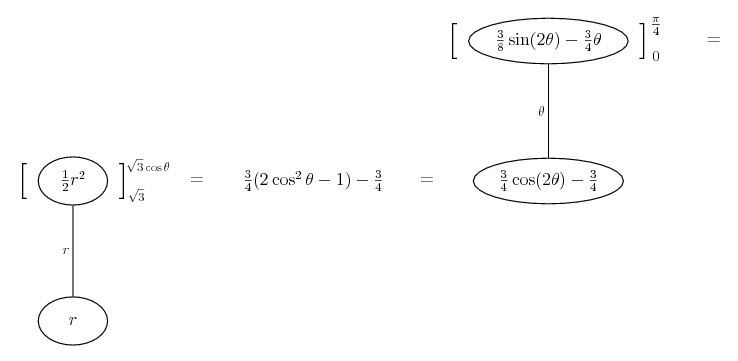# Thread: Evalute the iterated integral..two of them.

1. ## Evalute the iterated integral..two of them.

Alright I'm having some trouble with two of the problems in my homework.
Any help would be awesome.

1.$\displaystyle \int_{0}^\frac{\pi}{4}\int_{\sqrt{3}}^{\sqrt{3}\co s\theta}r dr d\theta$

2.$\displaystyle \int_{0}^\frac{\pi}{4}\int_{0}^{\cos\theta}3r^2\si n\theta drd\theta$

2. Just in case a picture helps...... where a straight line differentiates downwards (anti-differentiates up) with respect to the letter that labels it.

Spoiler:Spoiler:_________________________________________
Don't integrate - balloontegrate!

Balloon Calculus; standard integrals, derivatives and methods

Balloon Calculus Drawing with LaTeX and Asymptote!

3. $\displaystyle \int_{0}^\frac{\pi}{4}\int_{\sqrt{3}}^{\sqrt{3}\co s\theta}r dr \ d\theta = \frac{1}{2} \int^{\frac{\pi}{4}}_{0} (3 \cos ^{2} \theta -3) \ d\theta = -\frac{3}{2} \int^{\frac{\pi}{4}}_{0} \sin^{2} \theta \ d\theta$

$\displaystyle = -\frac{3}{2} \int^{\frac{\pi}{4}}_{0} \Big( \frac{1}{2} - \frac{\cos 2\theta}{2} \Big) \ d \theta = -\frac{3}{2} \Big( \frac{x}{2} - \frac{\sin 2 \theta}{4} \Big) \Big|^{\frac{\pi}{4}}_{0}$

$\displaystyle = \frac{-3}{2} \Big(\frac{\pi}{8} - \frac{1}{4}\Big) = \frac{3}{8} - \frac{3 \pi}{16}$

#### Search Tags

evalute, integraltwo, iterated